## A capacitor is connected to a 9 V battery and acquires a charge Q. What is the charge on the capacitor if it is connected instead to an 18 V

Question

A capacitor is connected to a 9 V battery and acquires a charge Q. What is the charge on the capacitor if it is connected instead to an 18 V battery? A capacitor is connected to a 9 V battery and acquires a charge Q. What is the charge on the capacitor if it is connected instead to an 18 V battery? 2Q 4Q Q Q/2 Request Answer Part B – Potential difference in a parallel-plate capacitor

in progress 0
5 months 2021-08-09T06:59:09+00:00 2 Answers 0 views 0

Part A: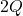Part B: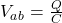Explanation:

The capacitance is given by: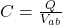Where: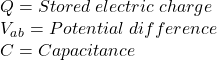So: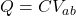In the first case: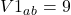And in the second case: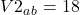or: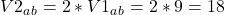C is a constant so: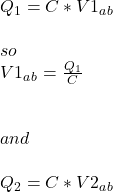Therefore: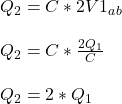Part B:

To find thepotential difference in a parallel-plate capacitor just isolate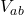from the capacitance equation:2Q

Explanation:

The expression for the electric charge stored in a capacitor is given as,

Q = CV………………… Equation 1

Where Q = Electric charge, C = Capacitance of the capacitor, V = Potential difference applied across the plates of the capacitor.

make C the subject of the equation

C = Q/V…………… Equation 2

Given: Q = Q, V = 9 V

Substitute into equation 2

C = Q/9 F.

If it is connected to an 18 V battery instead,

Q = CV

Given: C = Q/9 F, V = 18 V

Q = (Q/9)(18)

Q = 2Q.

Hence the charge on the capacitor = 2Q.